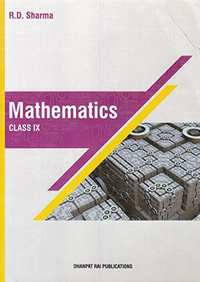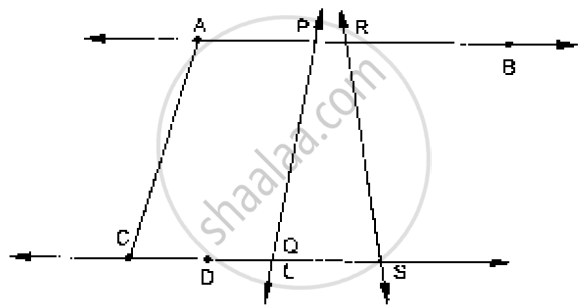# RD Sharma solutions for Mathematics for Class 9 chapter 9 - Introduction to Euclid’s Geometry [Latest edition]

#### Chapters## Solutions for Chapter 9: Introduction to Euclid’s Geometry

Below listed, you can find solutions for Chapter 9 of CBSE RD Sharma for Mathematics for Class 9.

Exercise 9.1Exercise 9.2
Exercise 9.1 [Page 8]

### RD Sharma solutions for Mathematics for Class 9 Chapter 9 Introduction to Euclid’s Geometry Exercise 9.1 [Page 8]

Exercise 9.1 | Q 1.1 | Page 8

Define the following terms:

Line segment

Exercise 9.1 | Q 1.2 | Page 8

Define the following term :

Collinear points

Exercise 9.1 | Q 1.3 | Page 8

Define the following terms :

Parallel lines

Exercise 9.1 | Q 1.4 | Page 8

Define the following term:

Intersecting lines

Exercise 9.1 | Q 1.5 | Page 8

Define the following term

Concurrent lines

Exercise 9.1 | Q 1.6 | Page 8

Define the following term

Ray

Exercise 9.1 | Q 1.7 | Page 8

Define the following term :

Half-line

Exercise 9.1 | Q 2.1 | Page 8

How many lines can pass through a given point?

Exercise 9.1 | Q 2.2 | Page 8

In how many points can two distinct lines at the most intersect?

Exercise 9.1 | Q 3.1 | Page 8

Given two points P and Q, find how many line segments do they deter-mine.

Exercise 9.1 | Q 3.2 | Page 8

Name the line segments determined by the three collinear points P, Q and R.

Exercise 9.1 | Q 4.01 | Page 8

Write the truth value (T/F) of each of the following statements:

Two lines intersect in a point.

Exercise 9.1 | Q 4.02 | Page 8

Write the truth value (T/F) of each of the following statements:

Two lines may intersect in two points

Exercise 9.1 | Q 4.03 | Page 8

Write the truth value (T/F) of each of the following statements

A segment has no length.

Exercise 9.1 | Q 4.04 | Page 8

Write the truth value (T/F) of each of the following statements:

Two distinct points always determine a line.

Exercise 9.1 | Q 4.05 | Page 8

Write the truth value (T/F) of each of the following statements

Every ray has a finite length.

Exercise 9.1 | Q 4.06 | Page 8

Write the truth value (T/F) of each of the following statements:

A ray has one end-point only.

Exercise 9.1 | Q 4.07 | Page 8

Write the truth value (T/F) of each of the following statement:

A segment has one end-point only.

Exercise 9.1 | Q 4.08 | Page 8

Write the truth value (T/F) of each of the following statements

The ray AB is same as ray BA.

Exercise 9.1 | Q 4.09 | Page 8

Write the truth value (T/F) of each of the following statement:

Only a single line may pass through a given point.

Exercise 9.1 | Q 4.1 | Page 8

Write the truth value (T/F) of each of the following statements:

Two lines are coincident if they have only one point in common.

Exercise 9.1 | Q 5 | Page 8

In the below fig., name the following:(i) five line segments.
(ii) Five rays.
(iii) Four collinear points.
(iv) Two pairs of non-intersecting line segments.

Exercise 9.1 | Q 6.1 | Page 8

Fill in the blank so as to make the following statement true:

Two distinct points in a plane determine a ________ line.

Exercise 9.1 | Q 6.2 | Page 8

Fill in the blank so as to make the following statement true:

Two distinct ________ in a plane cannot have more than one point in common.

Exercise 9.1 | Q 6.3 | Page 8

Fill in the blank so as to make the following statement

Geven a line and a point, not on the line, there is one and only         line pwahsiscehs through the given point and is       to the given line.

Exercise 9.1 | Q 6.4 | Page 8

Fill in the blank so as to make the following statement

A line separates a plane into                              parts namely the and the                  itself.

Exercise 9.2 [Page 9]

### RD Sharma solutions for Mathematics for Class 9 Chapter 9 Introduction to Euclid’s Geometry Exercise 9.2 [Page 9]

Exercise 9.2 | Q 1 | Page 9

How many least number of distinct points determine a unique line?

Exercise 9.2 | Q 2 | Page 9

How many lines can be drawn through both of the given points?

Exercise 9.2 | Q 3 | Page 9

How many lines can be drawn through a given point.

Exercise 9.2 | Q 4 | Page 9

In how many points two distinct lines can intersect?

Exercise 9.2 | Q 5 | Page 9

In how many points a line, not in a plane, can intersect the plane?

Exercise 9.2 | Q 6 | Page 9

In how many points two distinct planes can intersect?

Exercise 9.2 | Q 7 | Page 9

In how many lines two distinct planes can intersect?

Exercise 9.2 | Q 8 | Page 9

How many least number of distinct points determine a unique plane?

Exercise 9.2 | Q 9 | Page 9

Given three distinct points in a plane, how many lines can be drawn by joining them?

Exercise 9.2 | Q 10 | Page 9

How many planes can be made to pass through a line and a point not on the line?

Exercise 9.2 | Q 11 | Page 9

How many planes can be made to pass through two points?

Exercise 9.2 | Q 12 | Page 9

How many planes can be made to pass through three distinct points?

## Solutions for Chapter 9: Introduction to Euclid’s Geometry

Exercise 9.1Exercise 9.2## RD Sharma solutions for Mathematics for Class 9 chapter 9 - Introduction to Euclid’s Geometry

Shaalaa.com has the CBSE Mathematics Mathematics for Class 9 CBSE solutions in a manner that help students grasp basic concepts better and faster. The detailed, step-by-step solutions will help you understand the concepts better and clarify any confusion. RD Sharma solutions for Mathematics Mathematics for Class 9 CBSE 9 (Introduction to Euclid’s Geometry) include all questions with answers and detailed explanations. This will clear students' doubts about questions and improve their application skills while preparing for board exams.

Further, we at Shaalaa.com provide such solutions so students can prepare for written exams. RD Sharma textbook solutions can be a core help for self-study and provide excellent self-help guidance for students.

Concepts covered in Mathematics for Class 9 chapter 9 Introduction to Euclid’s Geometry are Concept for Euclid’S Geometry, Euclid’S Definitions, Axioms and Postulates, Equivalent Versions of Euclid’S Fifth Postulate.

Using RD Sharma Mathematics for Class 9 solutions Introduction to Euclid’s Geometry exercise by students is an easy way to prepare for the exams, as they involve solutions arranged chapter-wise and also page-wise. The questions involved in RD Sharma Solutions are essential questions that can be asked in the final exam. Maximum CBSE Mathematics for Class 9 students prefer RD Sharma Textbook Solutions to score more in exams.

Get the free view of Chapter 9, Introduction to Euclid’s Geometry Mathematics for Class 9 additional questions for Mathematics Mathematics for Class 9 CBSE, and you can use Shaalaa.com to keep it handy for your exam preparation.